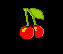# Lesson 25: Creating Random Values

### 25.1: Generating random numbers of an array in JavaScript

We have learned about the random generation of numbers in Javascript in Lesson 14. However, in this lesson, you will learn how to generate random numbers using an array. Since every element in an array is identified by a subscript, hence by randomizing the subscripts we will be able to generate random elements of the array.

We can generate randome numbers using the random method of the Math object. Math.random( ) generates numbers between 0 and 1. Therefore, to obtain random numbers between 0 and 6, we need to multiply Math.random( ) with 6, i.e Math.random( )*6. However, to obtain random integers between 0 and 6, we need to use the floor method of the Math object. The floor(x) method returns the greatest integer less than or equal to x. For example, Math.floor(5.4)=5

Equip with the above knowledge in mind, we shall proceed to write a subscript to simulate the spinning of a die that contains six faces and numbered 1, 2, 3,4,5,6.

#### Example 25.1 A Digital Dice

We can create a digital dice using the random and the floor methods, using the syntax as follows:

`Math.floor( Math.random( ) * 6 )`

It produces  random integers between 0 and 5, i.e 0,1,2,3,4,5. Thus,

`Numbers[ Math.floor( Math.random( ) * 6 ) ]`

produces random elements of the array with subscript 0,1,2,3,4 or 5, in this way we can generating random numbers 1 to 6, resembling the throwing of a die.

##### The JavaScript
```<script>
function spin(){
var Numbers = [ 1,2,3,4,5,6];
var myNumber=Numbers[Math.floor( Math.random() * 6 )] ;
document.getElementById("myNum").innerHTML=myNumber;

}
</script>
```

In addition, we also need to create a container to display the number and a button to trigger the JavaScript, as follows:

```    <div style="border:4px solid blue; width:100px; height:100px; text-align:center;padding:0"><h1 id="myNum" style="font-size:300%">1</h1></div>

<input type="button" onclick="spin()" value="Spin">
```

# 1

### 25.2: Generating random strings of an array

If an array is made up of strings or words, we can also generate random strings or words using the same reasoning. Each string is identified by a subscript, by randomizing the subscripts we can then generate random strings based on their subscripts.

The script is shown below:

```<script>
function choose(){
var fruits =[ "cherry", "grape", "orange"];
var myitem=fruits[Math.floor( Math.random() * 3 )] ;
document.getElementById("myfruit").innerHTML=myitem;

}
</script>
```

Click on the "Change" button to see what fruit you get.

The fruit is :

Click Example 25.2 to view the actual output

### 25.3 Generating random images using Array

Now we shall go a step further, generating random images using the same reasoning as above. Each image is identified by is subscript, therefore by randomizing the subscripts we can then create random images. To display the images, we just need to use the <img> tag.

The script is as follows:

```
<script>
var images =[ "cherry", "grape", "orange"];
var myitem=images[ Math.floor( Math.random() * 3 ) ] ;
var myimg = document.getElementById("myimage");
myimg.src=myitem+".gif";
</script>

```

You also need to create the button and the image container.

```<script img id="myimage" src="cherry.gif">
<script input type="button" onclick="change()" value="change">
```

Click the "change" button below to change the image.